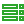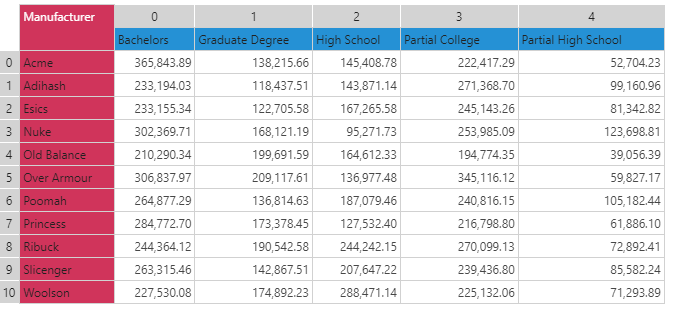#DataSetRow (PQL)

Returns a list of values found in a row of data in a given query result set.

• Returned Output: Numeric List
• Library: PQL \ Dynamic \ Row
• Version: 2018.00.000
• Compatibility: Any content (regardless of data source) in the relevant parts of the application

#### Syntax

DataSetRow()

* Click on the function's arguments above for more details on the input values.

• The row number is the zero-based index number of the row of data in the result set.

#### Examples

The examples below use the following example grid.This example returns the list of values from the "Poomah" row (264,877 to 105,182):

dataSetRow(6)

This example first finds the row with the lowest value using column 0 (lookupMinRow). Then, using that index, it returns the list of values from that row (210,290 to 39,056)

dataSetRow(lookupMinRow(0))